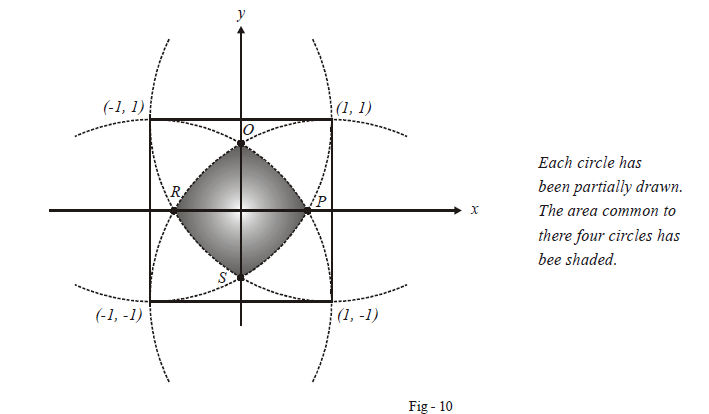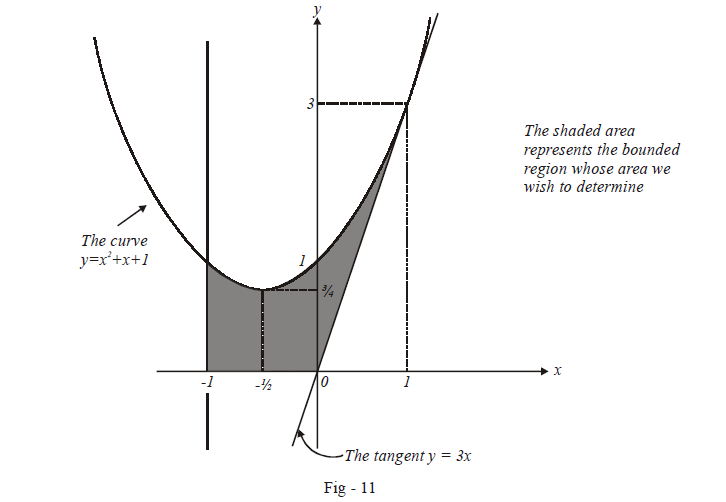# Problems On Areas Set-5

Go back to  'Areas and Volumes'

Example – 9

A square has its vertices at $$\left( {1,{\rm{ }}1} \right),{\rm{ }}\left( {1,{\rm{}}-1} \right),{\rm{ }}\left( {-1,{\rm{ }}-1} \right)\;{\rm{and}}\;{\rm{}}\left( {-1,{\rm{ }}1} \right).$$ Four circles of radius 2 are drawn, one centred at each vertex of the square. Find the area common to these four circles.

Solution:Let us evaluate the area in the first quadrant. For that, weneed to evaluate the $$x-$$co-ordinate of $$P$$. Notice that the circle centred at $$\left( {-1,{\rm{ }}-1} \right)$$ is the onewhich intersects the $$x-$$axis at $$P$$. The equation of this circle is given by:

\begin{align}&{\left( {x + 1} \right)^2} +{\left( {y + 1} \right)^2} = 4\\&\rm{when}\,\,y = 0,\,x = \sqrt 3 - 1 \qquad \qquad \dots (1)\\\end{align}

Thus           $$p \equiv \left( {\sqrt 3 - 1,0} \right)$$

The area in the first quadrant is now:

\begin{align}A_{1^{st}}&=\int\limits_0^{\sqrt {3 - 1} } {\left( {\sqrt {4 - {{\left( {x + 1}\right)}^2}} - 1} \right)dx} \qquad \qquad\left\{\begin{array}{l}\,\,{\rm{we\;used\;(1)\;to\;write\;down\;the\;equation\;}}\\\,{\rm{of\;the \;circle\;in\;an\;explicit\;form}}\end{array} \right\}\\&=\left\{ { - x + \frac{1}{2}\left( {x + 1} \right)\sqrt {4 - {{\left( {x + 1}\right)}^2}} + \frac{4}{2}} \right.{\sin ^{ - 1}}\left. {\left. {\left({\frac{{x + 1}}{2}} \right)} \right\}} \right|_0^{\sqrt {3 - 1}} \qquad \qquad {\rm{(verify\,this\,step)}}\\&= \left( { - \sqrt 3 + 1 + \frac{{\sqrt 3 }}{2} + 2{{\sin }^{ -1}}\frac{{\sqrt 3 }}{2}} \right) - \left( {\frac{{\sqrt 3 }}{2} + 2{{\sin }^{ -1}}\frac{1}{2}} \right)\\&= \frac{\pi }{3} - \sqrt 3\end{align}

Therefore, the total bounded area is

\begin{align}A&= 4 \times {A_{{1^{st}}}}\\&=4\left( {\frac{\pi }{3} - \sqrt 3 } \right)sq.\,\rm{units}\end{align}

Example – 10

Find the area of the region bounded by $$x + 1 = 0,y = {x^2} + x + 1$$ and the tangent to $$y = {x^2} + x + 1\,\,\,\rm{at}\,\,x = 1$$

Solution: Let us first determine the equation of thesaid tangent:

\begin{align}{\left. {\frac{{dy}}{{dx}}}\right|_{x = 1}} &= {\left. {\left( {2x + 1} \right)} \right|_{x = 1}}\\&= 3\end{align}

Also, when $$x = 1, y = 3$$

The required equation is

\begin{align}&y - 3 = 3\left( {x -1} \right)\\&\Rightarrow \quad y = 3x.\end{align}From the figure above, it should be clear that the area canbe calculated as described below:

\begin{align}A &= \int\limits_{ - 1}^0 {\left({{x^2} + x + 1} \right)} dx\, + \,\int\limits_0^1 {\left\{ {\left( {{x^2} + x +1} \right) - 3x} \right\}dx} \\& = \left. {\left( {\frac{{{x^3}}}{3} +\frac{{{x^2}}}{2} + x} \right)} \right|_{ - 1}^0\,\, + \left. {\left({\frac{{{x^3}}}{3} - {x^2} + x} \right)} \right|_0^1\\&= - \left({ - \frac{1}{3} + \frac{1}{2} - 1} \right)\,\, + \,\,\left. {\left({\frac{1}{3} - 1 + 1} \right)} \right|_0^1\\&=\frac{7}{6}\,\,sq.\,\rm{units}\end{align}